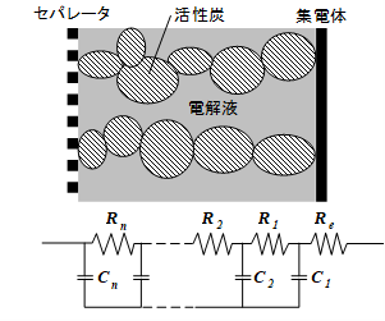### Supercapacitors Equivalent Circuit

Supercapacitors are composed of minute individual particles of activated carbon, and each of these has its own capacitance and resistance. Fig. 1 shows an equivalent circuit for a capacitor. The capacitance of the capacitor is the total capacitance of C1 and C2.[Fig. 1] Capacitor equivalent circuit

C1, C2: Capacitance of activated carbon electrodes
R1, R2: Insulation resistance
R3, R5: Electrode resistance
R4: Separator and electrolyte resistance

Total capacitance

Fig. 2 shows a more detailed equivalent circuit for each electrode.[Fig. 2] Capacitor equivalent circuit

As shown in Fig. 2, each individual minute particle of activated carbon acts as a capacitor with its own capacitance and resistance. Therefore, when the capacitor is charged, the particles (tiny capacitors) with less resistance charge first, followed by those with greater resistance. Moreover, if charging is stopped soon after reaching the charge voltage, the terminal voltage will drop as the particles with greater resistance are charged from those with less resistance (self-charging). Consequently, supercapacitors require "relaxation charging" (holding a constant voltage) after the charge voltage is reached in order to charge fully.
Tags
formula